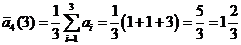## Moving Average

As the measurement sequence grows over time, sometimes we would like to get only the average of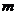last elements. This average is called moving-average (or movag in short) with kernel lengthcounted from the latest measurement or from the end of the sequence and denoted as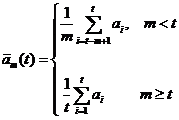(10)Diagram of moving average

If the kernel lengthlarger than the length of sequence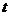, then we disregard the moving-length and count from the beginning of the sequence

Example

We have sequence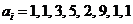. Let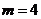and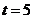then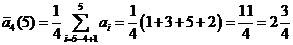Suppose we want to know the moving-average for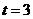, then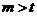and we have